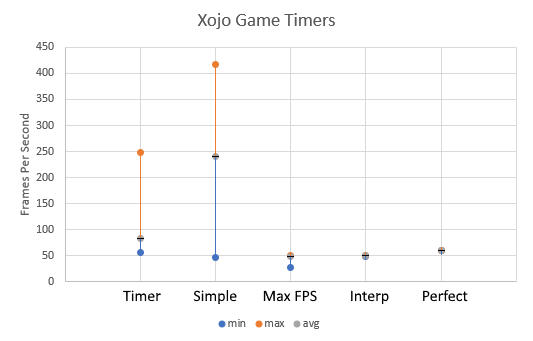This example shows how to create a simple function that adds two numbers together by creating a simple DLL in C# with Visual Studio 2019 that can be used in Xojo 2020 r1. The two major aspects are to: 1) Install the Microsoft Build Tools 2015, and 2) RGiesecke NuGet package.

*Uses Xojo API2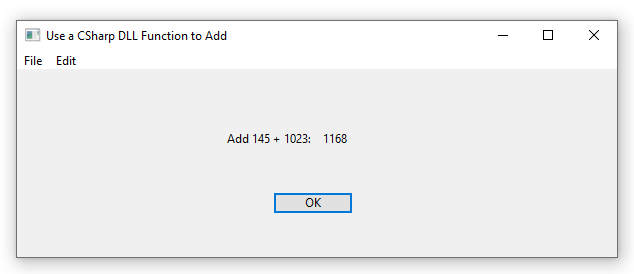Data in UTF form can be confusing, and adding endianness can be overwhelming. I have great news, as the Byte Order Marker can help remove this confusion when opening a file or receiving a file.

A byte order mark (BOM) are the hexadecimal numbers FE FF which are placed at the beginning of a file, or data stream, which are used to automatically determine the type of encoding of the data. It is common to write programs in many languages, and the way that non-english ASCII characters are shown is by using different encodings. Byte Order Mark should be invisible to the user, and the programs should automatically read this data and decode the text appropriately.

This example shows how to create a simple function that adds two numbers together by creating a simple DLL in C++ with Visual Studio 2019 that can be used in Xojo 2019 r1.1. The secret is that the functions will need to have "C"-like names to prevent name mangling. This example is for 64-bit applications.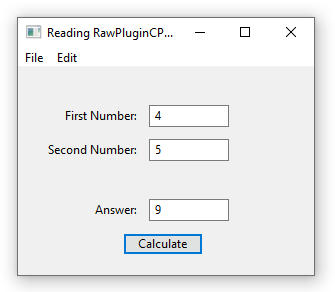Here are the steps to create the plugin in Visual Studio 2019 and read the DLL in Xojo.

Have you ever tried to draw a sine wave and it has been harder than what you thought? This example shows how to create a sine wave that can have its parameters changed at runtime and then the updated version of the wave can be seen in the program.

Figure 1. Screen grab of running program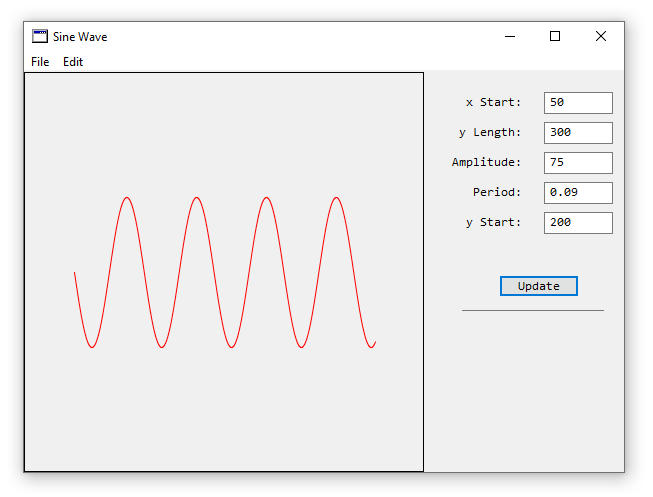The formula for generating a sine wave is y = A*sin(B(x-C))+D. Where A is the amplitude of the wave, B is the frequency of the wave, x is the x-axis value, C is the y-axis shift, and D is the vertical shift. This is good when calculating values on a piece of paper, and it becomes a little more challenging when doing this in a computer program.

This article is an adaptation of C# code to Xojo code and the C# article was originally written by Rod Stephens at  Draw a hexagonalgrid in C#. Rows and columns are numbered in a hexagonal grid when the rows start at zero at the top, and increase as you go near the bottom. Columns start at zero at the left and the value increases as you move to the right. Below is a screen grab of the hexagonal grid.

Figure 1. Hexagonal Grid Layout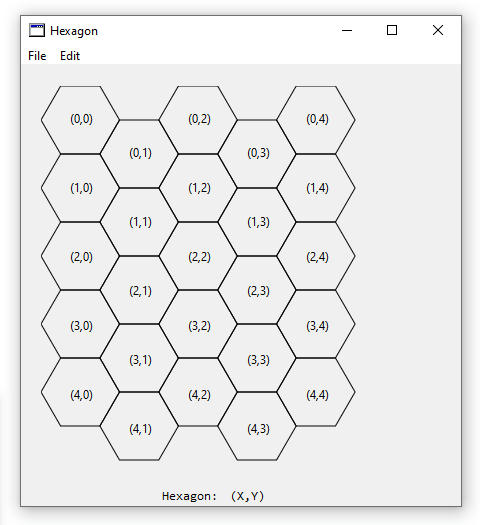Waves are difficult enough to generate, and how do you generate a wave that can be scaled? By scaling I mean that there is a constant number of amplitudes with a varying x-axis distance. This uses some of the code from the previous example at Make a Sine Wave. Scaling of a wave revolves around a modification of the code where the period is equal to the inverse of the frequency (period = 1/frequency), which has been changed in the program.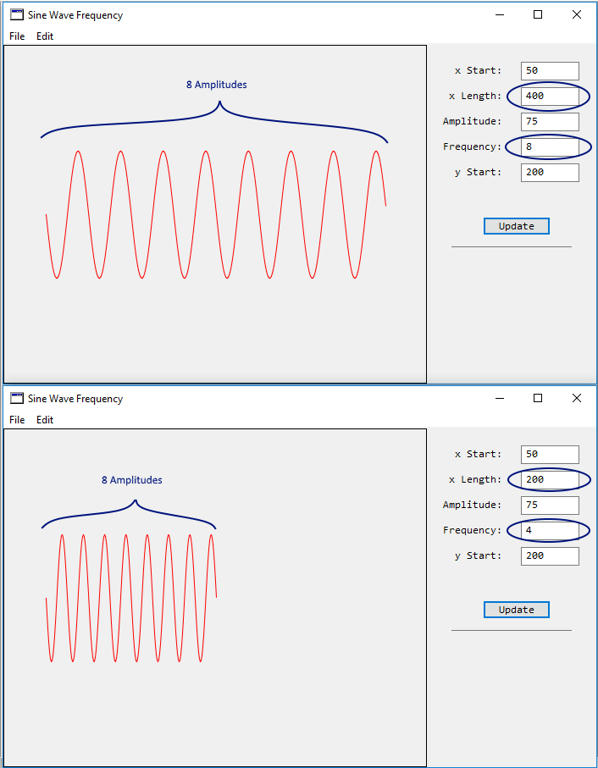As simple as it sounds, drawing an arrow with an arrowhead, that can change colour and thickness of the lines can be challenging. The code is relatively easy, and it’s the mathematics that makes it complicated to look right. I created a method that makes it easy and is portable to use in your program. Code can be downloaded for Xojo 2019 r1.1 on GitHub at: https://github.com/eugenedakin/XojoArrow

*Uses Xojo API1

Below is a screen grab when drawing one coloured arrow that starts at x:y coordinate (5,5) and the arrowtip is a coordinate (100,100). Thickness of the line is set to 2, and the colour is &c11CCDD. Below is a screen grab of the running program with a Canvas.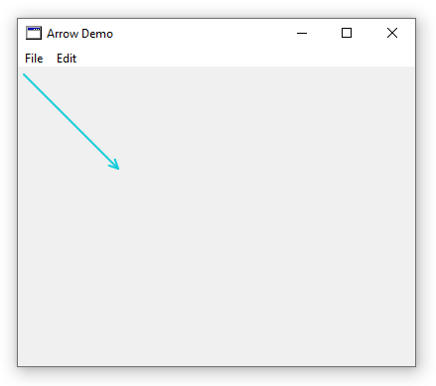With the new Xojo 2020 r2 version, there have been a few changes for 0-based drawing and using the GraphicsPath technique. This example incorporates both changes into a modified method to work with these changes, and the output is shown below.

*Uses Xojo API2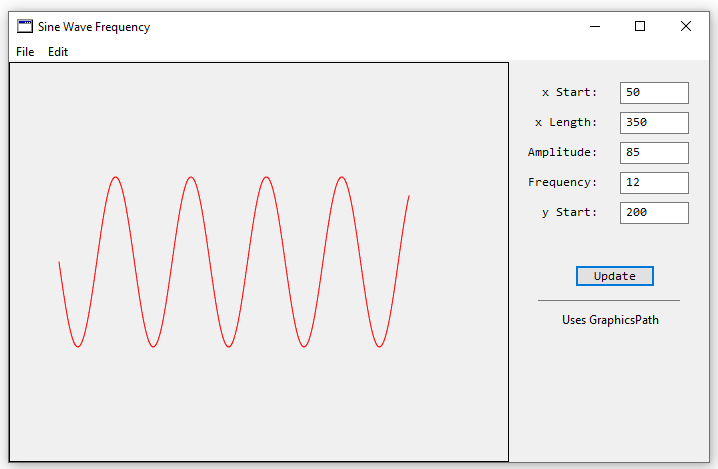This example shows how to use various declares and Xojo API2 code to dynamically retrieve the width and height of a screen so that these dimensions can be used to resize your main window.

*Uses Xojo API2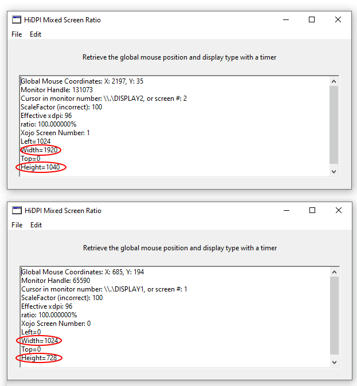The loop to run games is the main and most-important loop, as the game will not run without it. There are not many articles on Game Loops with the Desktop with Xojo. I will talk about four different types of game loops, and will provide the pros and cons of these game loops and will then provide the best one (in my humble opinion) to use. This article will involve a little of the math(s) topic, so let’s dig in shall we!

Four types of loops to run games are: 1) Timer, 2) Simple Game Loop, 3) Minimum Time Loop (Maximum FPS), and 4) Minimum Time and Interpolation (Best at the moment).

The loops will be measured in microseconds, which means that 1,000,000 microseconds is equal to 1 second. For the below examples, the chosen frame rate is 60 frames-per-second (FPS), which means that the loop should draw equally, and every time, at 16,666 microseconds (1,000,000 microseconds/60 FPS).